### 13.1.3.1 Pushing a box

Imagine using a differential drive robot to push a box around on the floor, as shown in Figure 13.7a. It is assumed that the box is a convex polygon, one edge of which contacts the front of the robot. There are frictional contacts between the box and floor and also between the box and robot. Suppose that the robot is moving slowly enough so that dynamics are insignificant. It is assumed that the box cannot move unless the robot is moving. This prohibits manipulations such as kicking'' the box across the room. The term stable pushing [12,671,681] refers to the case in which the robot moves the box as if the box were rigidly attached to the robot.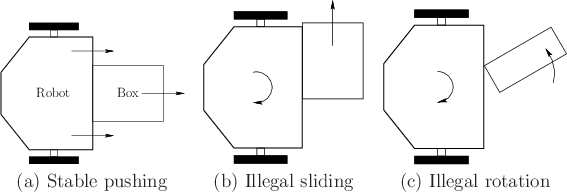As the robot pushes the box, the box may slide or rotate, as shown in Figures 13.7b and 13.7c, respectively. These cases are considered illegal because they do not constitute stable pushing. What motions of the robot are possible? Begin with the configuration transition equation of the differential drive robot, and then determine which constraints need to be placed on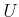to maintain stable pushing. Suppose that (13.17) is used. It is clear that only forward motion is possible because the robot immediately breaks contact with the box if the robot moves in the opposite direction. Thus,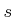must be positive (also, to fit the quasi-static model,should be small enough so that dynamical effects become insignificant). How should the rotation rate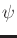be constrained? Constraints ondepend on the friction model (e.g., Coulomb), the shape of the box, and the particular edge that is being pushed. Details on these constraints are given in [671,681]. This leads to an interval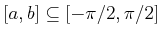, in which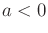and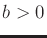, and it is required that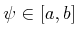. This combination of constraints produces a motion model that is similar to the Dubins car. The main difference is that the maximum steering angle in the left and right directions may be different.

To apply this model for planning, it seems that the C-space should be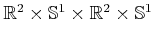because there are two rigid bodies. The manipulation planning framework of Section 7.3.2 can be applied to obtain a hybrid system and manipulation graph that expresses the various ways in which the robot can contact the box or fail to contact the box. For example, the robot may be able to push the box along one of several possible edges. If the robot becomes stuck, it can change the pushing edge to move the box in a new direction.

Steven M LaValle 2020-08-14